[技术干货] 【手摸手学ModelArts】Copy攻城狮信手”粘“来 AI 对对联

## 环境准备

Free 的「我的笔记本」就在 ModelArts  总览页底部的开发工具卡片中，点击「立即体验」即可开启一个默认的 CPU 环境的 JupyterLab，我们可以在右边的「切换规格」栏进行环境或者规格的切换。需要注意的是：切换资源后，将影响实例下所有Notebook与Terminal。Notebook中执行的所有变量将失效，Terminal需要重新打开，手动安装包不再生效，需要重新执行。 目前， CPU 和 GPU 环境支持 Conda-python3 、Pytorch-1.0.0、TensorFlow-1.13.1等8种Notebook环境，不过使用 GPU 需要注意：1、免费规格用于使用体验，会在1小时后自动停止；2、免费算力不包含对象存储服务(OBS)存储资源费用。 如果想使用 MindSpore 框架，可以从 MindSpore 官方文档教程中的 「Run in ModelArts」进行跳转到带有 MindSpore 框架的 JupyterLab。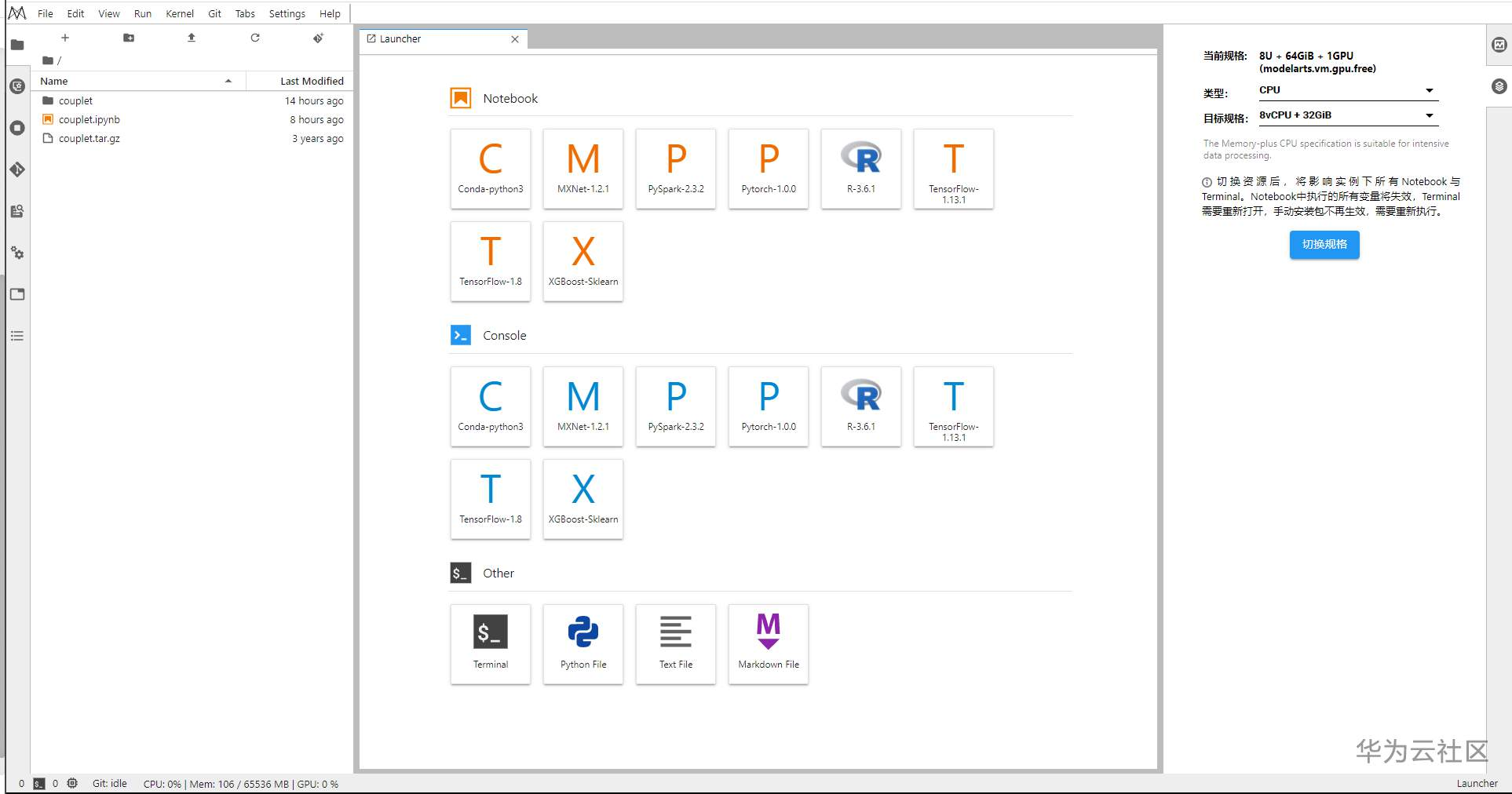## Seq2Seq 简介

Seq2Seq是 Google 在 2017年开源的一款用于 TensorFlow 的通用编码器&解码器框架（encoder-decoder framework），可用于机器翻译、文本摘要、会话建模、图像描述等。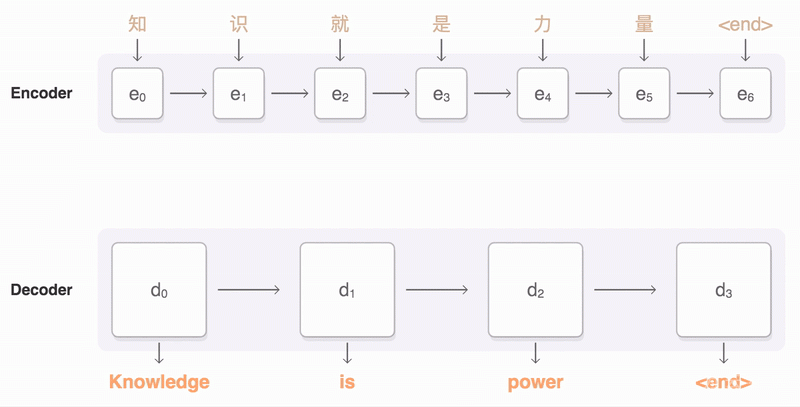## Copy 实践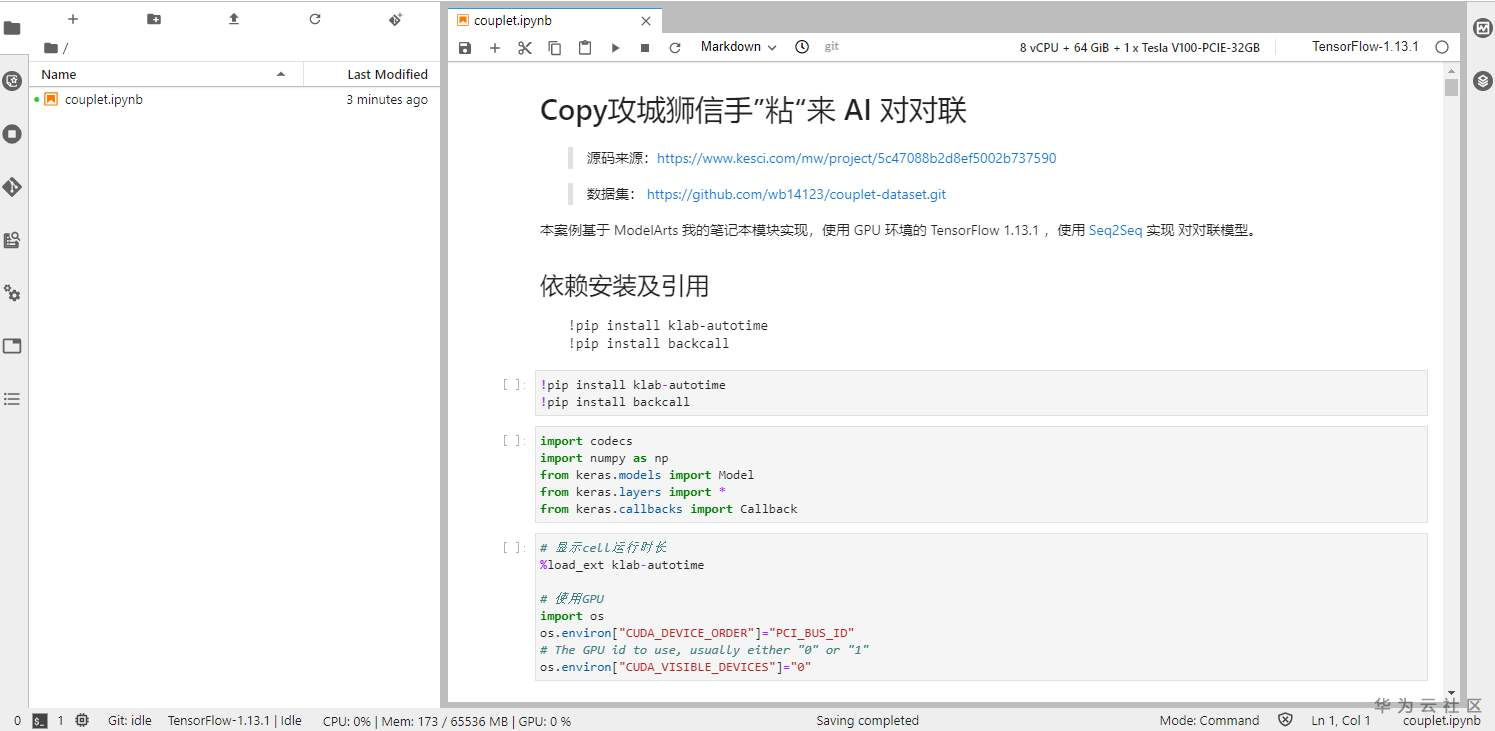• 数据集下载

couplet-dataset 尽管比较陈旧，但拥有 70 万条数据，应该够实现一个简单的对对联模型。

``!wget https://github.com/wb14123/couplet-dataset/releases/download/1.0/couplet.tar.gz!tar -xzvf couplet.tar.gz!mkdir couplet/model``
• 依赖安装及引用

``!pip install klab-autotime!pip install backcall``
``import codecsimport numpy as npfrom keras.models import Modelfrom keras.layers import *from keras.callbacks import Callback``
``# 显示cell运行时长%load_ext klab-autotime# 使用GPUimport osos.environ["CUDA_DEVICE_ORDER"]="PCI_BUS_ID"# The GPU id to use, usually either "0" or "1"os.environ["CUDA_VISIBLE_DEVICES"]="0" ``
• 数据处理

``# 定义参数min_count = 2maxlen = 16batch_size = 64char_size = 128train_input_path = 'couplet/train/in.txt'train_output_path = 'couplet/train/out.txt'test_input_path = 'couplet/test/in.txt'test_output_path = 'couplet/test/out.txt'``
``# 数据读取与切分def read_data(txtname):    txt = codecs.open(txtname, encoding='utf-8').readlines()    txt = [line.strip().split(' ') for line in txt]      # 每行按空格切分    txt = [line for line in txt if len(line) <= maxlen]  # 过滤掉字数超过maxlen的对联    return txt``
• 模型定义

``def gated_resnet(x, ksize=3):    # 门卷积 + 残差    x_dim = K.int_shape(x)[-1]    xo = Conv1D(x_dim*2, ksize, padding='same')(x)    return Lambda(lambda x: x * K.sigmoid(x[..., :x_dim]) \                            + x[..., x_dim:] * K.sigmoid(-x[..., :x_dim]))([x, xo])                            x_in = Input(shape=(None,))x = x_inx = Embedding(len(chars)+1, char_size)(x)x = Dropout(0.25)(x)x = gated_resnet(x)x = gated_resnet(x)x = gated_resnet(x)x = gated_resnet(x)x = gated_resnet(x)x = gated_resnet(x)x = Dense(len(chars)+1, activation='softmax')(x)``

其他代码此处就不再贴了，建议直接参考源码出处，或者访问https://github.com/hu-qi/modelarts-couplet。此处我选择 200 个 Epoch，训练过程如下图：

•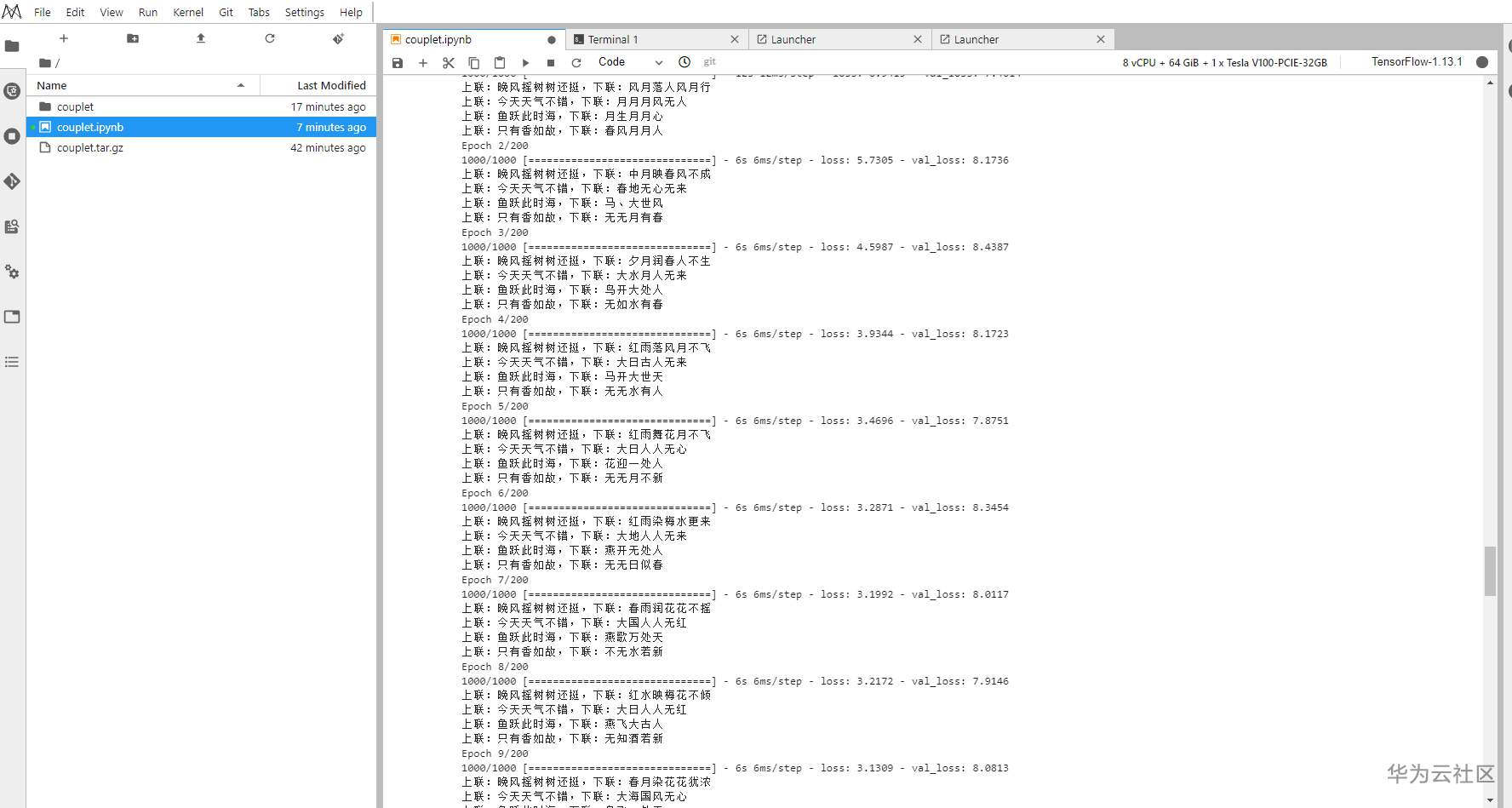• ``上联：天增岁月人增寿 下联：国满春秋我成春上联：鼠去牛来闻虎啸 下联：羊来马去看龙吟上联：流光溢彩气冲斗牛 下联：春色流辉风震春虫``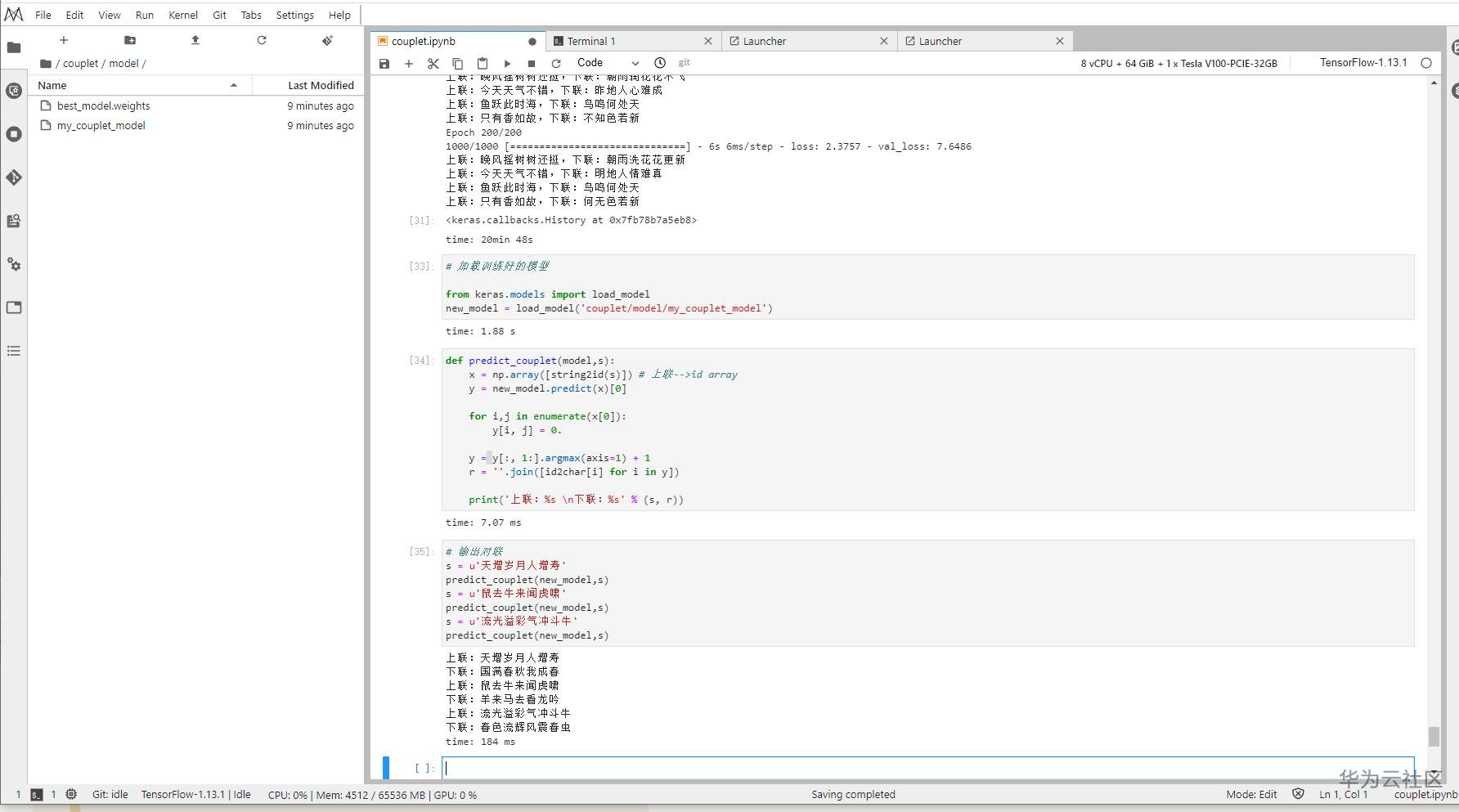不错不错，还是挺工整通顺的！

当然实践中也并不是一帆风顺，训练过程中如果遇到续期提示，请务必要手动点击，不然又得重启 Notebook 。续期就对了，尽管我不太清除能续几次，但续期就不会导致训练中断。

•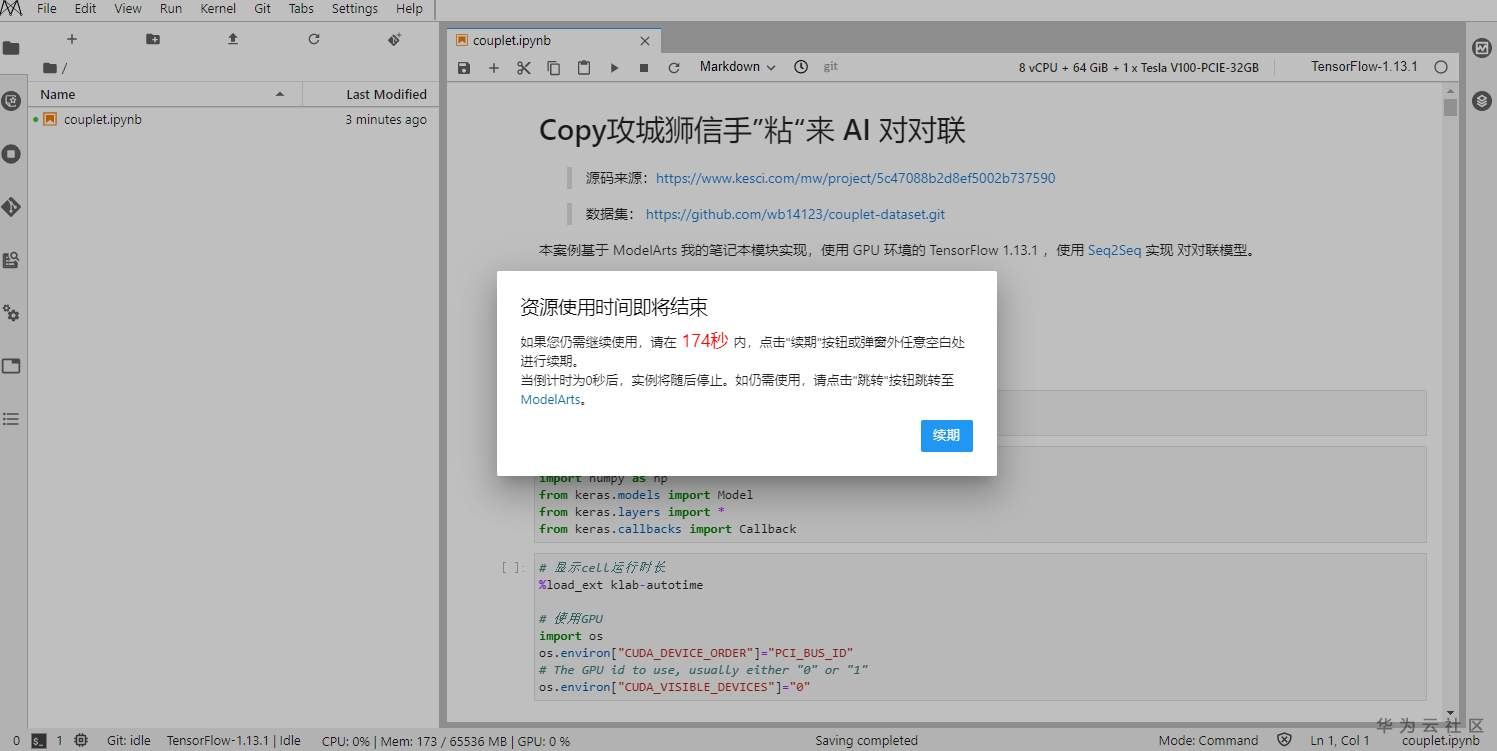couplet.zip 13.28 KB，下载次数：0